# Tutorial 7: Conditional Proof

Logical System

2013

### Skills to be acquired:

Learning conditional proof.

Bergmann The Logic Book Section 5.1 and 5.4

### The Tutorial:

The five remaining sentential rules of inference are slightly more difficult than the ones that we have met before. They are slightly more difficult in that they require you to make new assumptions, and the correct new assumptions at that. However they follow a similar pattern to each other so mastery of one should lead to mastery of the others.

Two of them are classical forms of inference, dating back thousands of years-- we will look at these first.

Conditional proof is a technique for proving statements of the form 'If A, then B' and it procedes by first assuming A, then deriving B, and finally dropping the assumption of A to conclude 'If A, then B'. (This technique is commonplace in mathematics. Many mathematical theorems have the right form (for example, 'If a triangle is Euclidean, then its angles sum to 180 degrees'), and such theorems are proved in the manner indicated (for example, 'Assume that the triangle is Euclidean, ... )).

In our rule system conditional proof appears as the Rule for the Introduction of the Conditional ⊃I. This, then, is a rule which gets used when you are trying to obtain a conditional formula.

The circumstance it gets invoked looks like this.

 ? ? << G⊃F ?

That is, the formula you are trying to obtain has a ⊃ as its main connective-- the formula you are trying to obtain has the form <if-clause> ⊃ <then-clause>. In the illustration, for example, G is the <if-clause> and F is the <then-clause> .

You follow a three stage process. First you assume the <if-clause>. The illustrated fragment will then become

 G Ass ? ?<< G⊃F ?

Second you continue deriving in this new context until you manage to derive the <then-clause> thus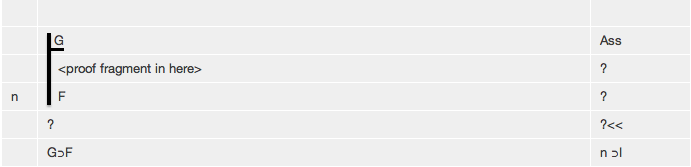Finally you select the <then-clause> and click ⊃I to complete the conditional proof.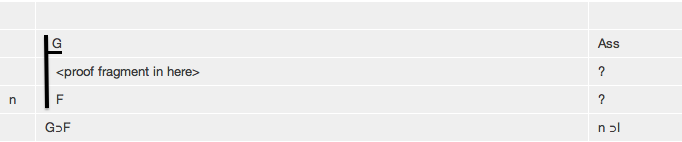### Notation

The authors of The Logic Book use a particular kind of notation in connection with Conditional Proof (and with Reductio and v Elimination, which we will meet later). For example, they notate a conditional proof of ∴(G⊃(G&G)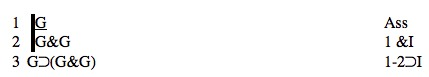Deriver will notate the same proof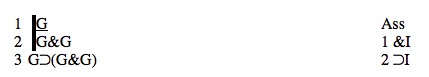The focus here is on the justification of line 3. They write '1-2 ⊃I', Deriver will write '2 ⊃I'. They refer to all the lines of a 'sub-proof' whereas Deriver refers only to the last line. Now, The Logic Book uses as its intellectual foundation Gentzen Sequent Calculus (see p162 note). And in the Gentzen Sequent Calculus every line, every sequent, is a valid argument in its own right. (And it is important that students understand this-- this point is explained in Tutorial 6.) And correct application of a Rule like ⊃I depends on only one other line, one other sequent. In the above example, line 3 depends only on line 2, it does not depend on line 1. So the The Logic Book uses a mildly misleading notation. Deriver does not do this.

## Exercises to accompany Tutorial 7

### Exercise 1 (of 3)

After starting the derivation switch on Tactics (under the Wizard Menu in the Proof Panel) and derive the following. Start by selecting the Rule ⊃I to lay out the derivation properly. Notice the form of each derivation-- the <if-clause> gets taken as a new assumption, from it the <then-clause> is derived, and the new assumption is dropped to conclude

<if-clause> ⊃ <then-clause>

a) (F⊃G), (G⊃H) ∴ (F⊃H)
b) (F⊃(G⊃H)), (F⊃G) ∴ (F⊃H)
c) (F⊃G) ∴ (F⊃(F&G))

### Exercise 2 (of 3)

Now try to do the same derivations without using Tactics. To do each of them the first move is to assume the <if-clause> and in each case here that is F. To make an assumption you choose Assumption off the Rules Menu. When you have derived the <then-clause> select it and click ⊃I. (*There are some technical terms that appear here: the Rule of Assumption is called 'TI' (short for Trival Inference), and the new assumption is called an 'Antecedent'*)

a) (F⊃G), (G⊃H) ∴ (F⊃H)

(*this requires you to assume F and try to derive H as the last line of a subproof*)

b) (F⊃(G⊃H)), (F⊃G) ∴ (F⊃H)

(*this requires you to assume F and try to derive H as the last line of a subproof*)

c) (F⊃G) ∴ (F⊃(F&G))

(*this requires you to assume F and try to derive F&G as the last line of a subproof*)

### Exercise 3 (of 3)

There are a number of important theorems which can be expressed as equivalences-- here we consider slightly weakened versions of them which are conditionals.

Derive (with or without Tactics)

Commutation

a) ∴ (F&G)⊃(G&F)

Double Negation

b) ∴ ∼∼F⊃F

Exportation

c) ∴ (F⊃(G⊃H))⊃((F&G)⊃H)
d) ∴ ((F&G)⊃H)⊃(F⊃(G⊃H))

[The next, somewhat tangential remarks, may or may not apply. It relates to menus being enabled and disabled. In the past, they have had that feature, but they do not right now and may not going forward. So likely you can skip the next remarks.

The last derivation ∴ ((F&G)⊃H)⊃(F⊃(G⊃H)) brings up an interesting point. If you do it by Tactics , part way through you can end up like this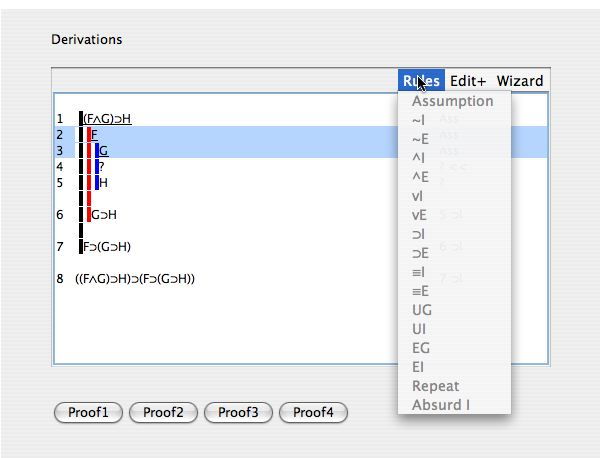Now, you are trying to get (F&G) from lines 2 and 3 (to use to get H from line 1), but, on the Rules Menu And Introduction &I is not enabled! What to do? Well, what is happening here is that Tactics is switched on, and ∧I is enabled only if the target line is an 'And' (and line 5, H, does not have 'And' as its main connective). You have a choice here. The timid way is to switch Tactics off and do the step (and switch Tactics back on, if you wish). The bolder way is to use New Sub Goal (under Edit) and enter (F&G) as the New Sub Goal. There is a Help movie showing this: New Sub Goal. ]

If you decide to use the web application for the exercises you can launch it from here Deriver[Bergmann] — username 'logic' password 'logic'. Then either copy and paste the above formulas into the Journal or use the Deriver File Menu to Open Web Page with this address https://softoption.us/test/easyDeriver/CombinedExercisesEasyDBergmann.html .

Preferences

You may need to set some Preferences for this.

• you can check that the parser is set to bergmann.